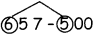Tamilnadu State Board New Syllabus Samacheer Kalvi 4th Maths Guide Pdf Term 3 Chapter 2 Numbers Ex 2.6 Textbook Questions and Answers, Notes.

## Tamilnadu Samacheer Kalvi 4th Maths Solutions Term 3 Chapter 2 Numbers Ex 2.6

Add and subtract the following problems using multiples of 10, 100 (Mentally)

Question 1.
745 + 40 = _______
Hint: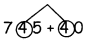4 + 4 = 8
745 + 40 = 785
785Question 2.
328 + 30 = _______
Hint: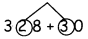2 + 3 = 5
328 + 30 = 358
358

Question 3.
566 + 20 = _______
Hint: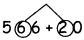6 + 2 = 8
566 + 20 = 586
586Question 4.
475 + 100 = _______
Hint: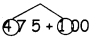4 + 1 = 5
475 + 100 = 575
575

Question 5.
686 + 300 = _______
Hint: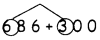6 + 3 = 9
686 + 300 = 986
986Question 6.
345 + 600 = _______
Hint: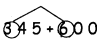3 + 6 = 9
345 + 600 = 945
945

Question 7.
6348 – 10 = _______
Hint: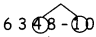4 – 1 = 3
6348 – 10 = 6338
6338Question 8.
541 – 40 = _______
Hint: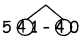4 – 4 = 0
541 – 40 = 501
501

Question 9.
495 – 300 = _______
Hint: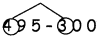4 – 3 = 1
495 – 300 = 195
195Question 10.
657 – 500 = _______
Hint: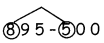6 – 5 = 1
657 – 500 = 157
157

Question 11.
895 – 500 = _______
Hint: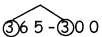8 – 5 = 3
895 – 500 = 395